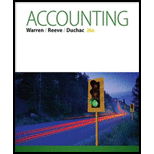Chapter 1, Problem 1.2BPE### Accounting (Text Only)

26th Edition
Carl Warren + 2 others
ISBN: 9781285743615

#### Solutions

Chapter
Section### Accounting (Text Only)

26th Edition
Carl Warren + 2 others
ISBN: 9781285743615
Textbook Problem
3 views

# Accounting equationFritz Evans is the owner and operator of Be-The-One, a motivational consulting business. At the end of its accounting period, December 31, 2015, Be-The-One has assets of $395,000 and liabilities of$97,000. Using the accounting equation, determine the following amounts: a. Owner’s equity as of December 31, 2015. b. Owner’s equity as of December 31, 2016, assuming that assets decreased by $65,000 and liabilities increased by$36,000 during 2016.

(a)

To determine

Accounting equation

Accounting equation is an accounting tool expressed in the form of equation, by creating a relation between resources or assets of a company and claims of resources by creditors and owners. Accounting equation is expressed as shown below:

Assets = Liabilities + Owners' Equity

To solve: For owners’ equity as of December 31, 2015, using accounting equation

Explanation

Solve for owners’ equity using accounting equation, if total assets is $395,000, and total liabilities is$97,000.

Assets = Liabilities + Owners' Equity$395,000 =$97,000 + Owners' Equity</

(b)

To determine

To solve: For owners’ equity as of December 31, 2016, using accounting equation

### Still sussing out bartleby?

Check out a sample textbook solution.

See a sample solution

#### The Solution to Your Study Problems

Bartleby provides explanations to thousands of textbook problems written by our experts, many with advanced degrees!

Get Started

#### Find more solutions based on key concepts